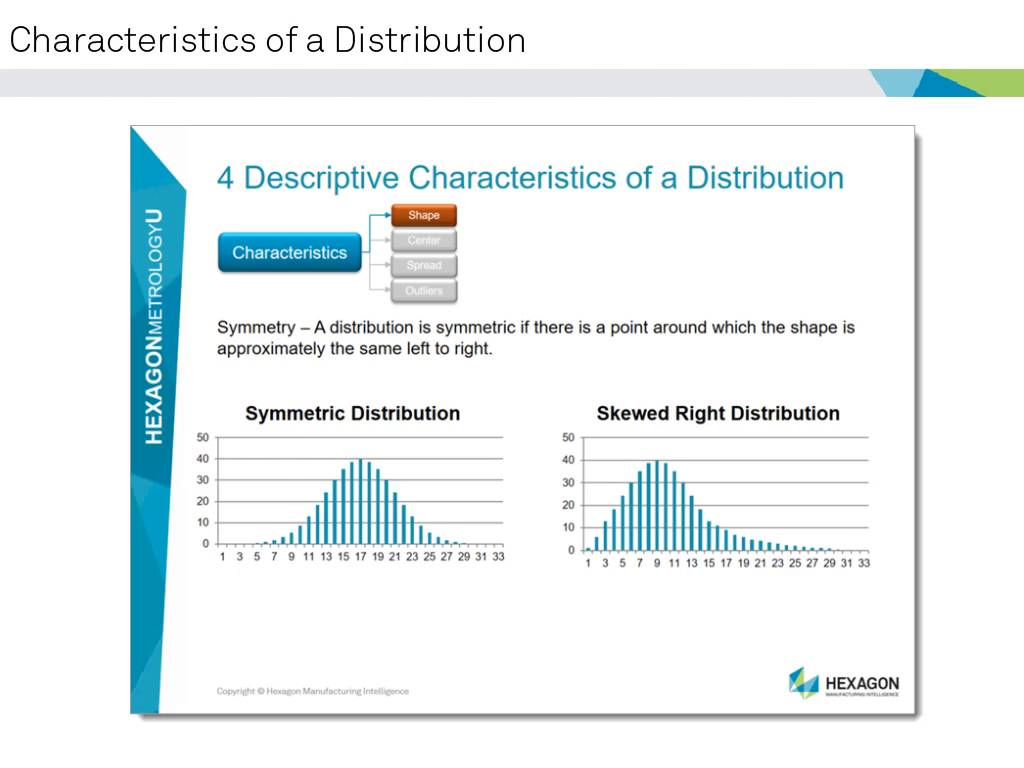You are not logged in.Language:Statistics 101 [Exploratory Data Analysis] (11040-11-10)

\$49.00 (USD)

This online eLearning introduction covers the basic ideas of distributions, charting, and basic numerical values. Concepts such as the Histogram, Mean value of a distribution and Standard deviation of a distribution are covered in this introductory course.Estimated Length: 2 hour(s)

## Lessons

Name View Schedule1. Introduction, Data, Datasets and Distributions -2. Center, Spread & Box Plot -3. Normal Distribution -4. Online Class Survey -